Sunday, August 9, 2020
Home > CBSE Class 12 > NCERT Solutions for Class 12 Maths Continuity and Differentiability Exercise 5.3

# NCERT Solutions for Class 12 Maths Continuity and Differentiability Exercise 5.3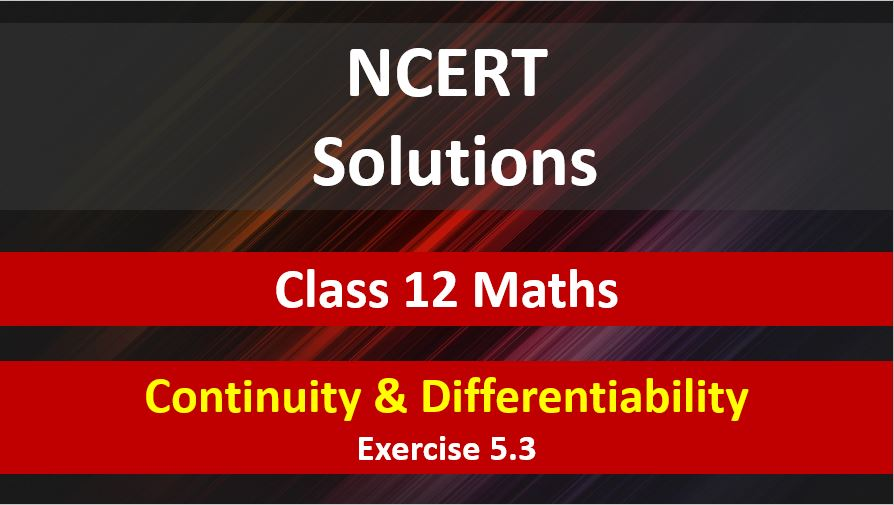# NCERT Solutions for Class 12 Maths Continuity and Differentiability

Hi Students, Welcome to Amans Maths Blogs (AMB). In this post, you will get the NCERT Solutions for Class 12 Maths Continuity and Differentiability Exercise 5.3. This NCERT Solutions can be downloaded in PDF file. The downloading link is given at last.

CBSE Class 12th is an important school class in your life as you take some serious decision about your career. And out of all subjects, Maths is an important and core subjects. So CBSE NCERT Solutions for Class 12th Maths is major role in your exam preparation as it has detailed chapter wise solutions for all exercise.

NCERT Solutions for Class 12 Maths are not only the solutions of Maths exercise but it builds your foundation of other important subjects. Getting knowledge of depth concept of CBSE Class 12th Maths like Algebra, Calculus, Trigonometry, Coordinate Geometry help you to understand the concept of Physics and Physical Chemistry.

As we know that all the schools affiliated from CBSE follow the NCERT books for all subjects. You can check the CBSE NCERT Syllabus. Thus, NCERT Solutions helps the students to solve the exercise questions as given in NCERT Books.

## NCERT Solutions for Class 12 Maths Continuity and Differentiability Exercise 5.3

Find dy/dx in the following

NCERT Solutions for Class 12 Maths Continuity and Differentiability Exercise 5.3: Ques No 1.

2x + 3y = sin x

NCERT Solutions:

We are given that 2x + 3y = sin x.

Differentiating both sides with respect to x, we getNCERT Solutions for Class 12 Maths Continuity and Differentiability Exercise 5.3: Ques No 2.

2x + 3y = sin y

NCERT Solutions:

We are given that 2x + 3y = sin y.

Differentiating both sides with respect to x, we getNCERT Solutions for Class 12 Maths Continuity and Differentiability Exercise 5.3: Ques No 3.

ax + by2 = cos y

NCERT Solutions:

We are given that ax + by2 = cos y.

Differentiating both sides with respect to x, we getNCERT Solutions for Class 12 Maths Continuity and Differentiability Exercise 5.3: Ques No 4.

xy + y2 = tan x + y

NCERT Solutions:

We are given that xy + y2 = tan x + y.

Differentiating both sides with respect to x, we getNCERT Solutions for Class 12 Maths Continuity and Differentiability Exercise 5.3: Ques No 5.

x2 + xy + y2 = 100

NCERT Solutions:

We are given that x2 + xy + y2 = 100.

Differentiating both sides with respect to x, we get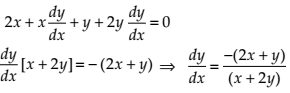NCERT Solutions for Class 12 Maths Continuity and Differentiability Exercise 5.3: Ques No 6.

x3 + x2y + xy2 + y3 = 81

NCERT Solutions:

We are given that x3 + x2y + xy2 + y3 = 81.

Differentiating both sides with respect to x, we get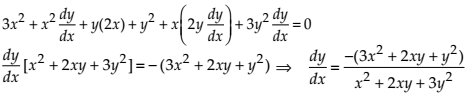NCERT Solutions for Class 12 Maths Continuity and Differentiability Exercise 5.3: Ques No 7.

sin2 y + cos xy = π

NCERT Solutions:

We are given that sin2 y + cos xy = π.

Differentiating both sides with respect to x, we getNCERT Solutions for Class 12 Maths Continuity and Differentiability Exercise 5.3: Ques No 8.

sin2 x + cos2 y = 1

NCERT Solutions:

We are given that sin2 x + cos2 y = 1.

Differentiating both sides with respect to x, we getNCERT Solutions for Class 12 Maths Continuity and Differentiability Exercise 5.3: Ques No 9.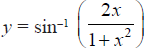NCERT Solutions:

We are given that.NCERT Solutions for Class 12 Maths Continuity and Differentiability Exercise 5.3: Ques No 10.NCERT Solutions:

We are given that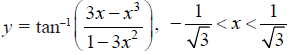.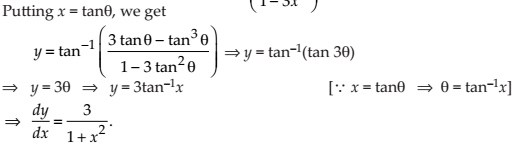NCERT Solutions for Class 12 Maths Continuity and Differentiability Exercise 5.3: Ques No 11.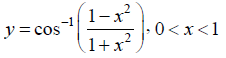NCERT Solutions:

We are given that.NCERT Solutions for Class 12 Maths Continuity and Differentiability Exercise 5.3: Ques No 12.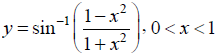NCERT Solutions:

We are given that.NCERT Solutions for Class 12 Maths Continuity and Differentiability Exercise 5.3: Ques No 13.NCERT Solutions:

We are given that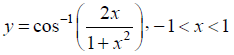.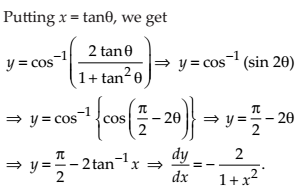NCERT Solutions for Class 12 Maths Continuity and Differentiability Exercise 5.3: Ques No 14.NCERT Solutions:

We are given that.NCERT Solutions for Class 12 Maths Continuity and Differentiability Exercise 5.3: Ques No 15.NCERT Solutions:

We are given that.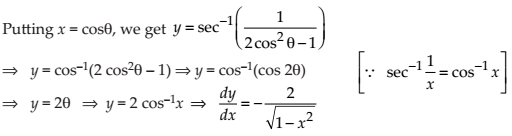error: Content is protected !!